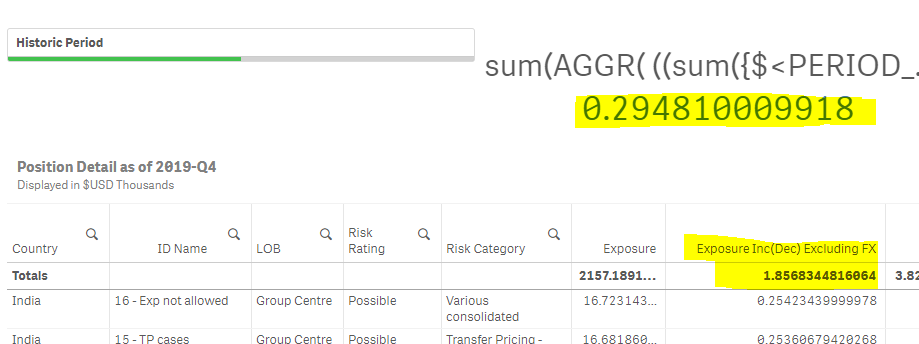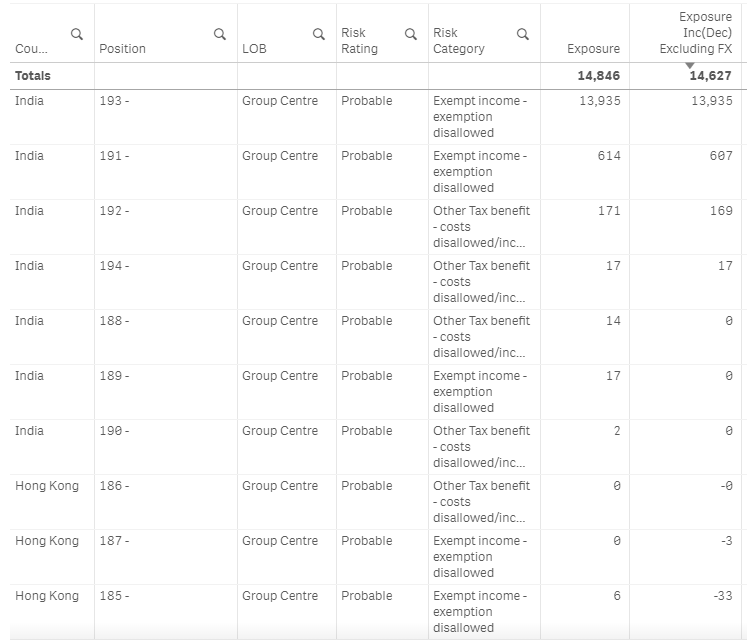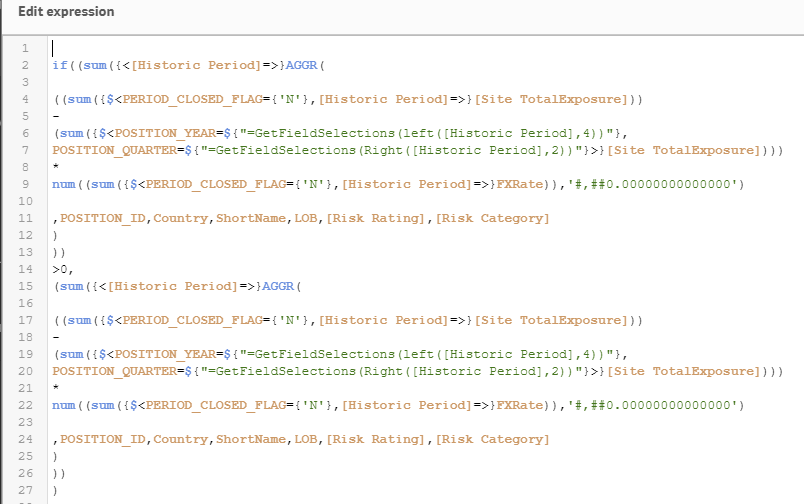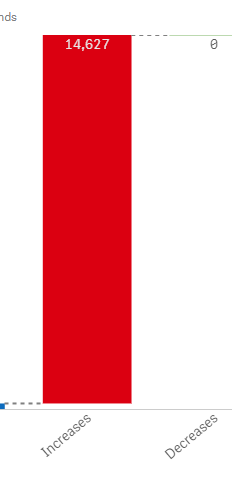# New to Qlik Sense

If you’re new to Qlik Sense, start with this Discussion Board and get up-to-speed quickly.

Announcements
Become an analytics expert with Qlik's new 15 week course: Applied Data Analytics using Qlik Sense. READ MORE
cancel
Showing results for
Did you mean:Contributor

## Totals function of expression as "sum" in a table to be shown in KPI in QlikSense

Hi All,

I have a straight table in which i am using a measure expression and using Sum as the dropdown from Totals Function. I have to show the same expression in the KPI object but the KPI object does not have the option to select the Totals Function explicitly.So, i am using sum(Aggr() on the basis of dimension present in the filter.But still the values are not matching if i select one of the filter "Historic Period".

This expression used in the table contains a sub part of set-analysis which has [Historic Period]=> condition so that the values does not change on the filter selection.Please note this filter is not present as dimension in the chart.Still i added it in the dimension of SUM(AGGR() expression but then it shows the value of the KPI object as 0 .

Expression used in Table:

((sum({\$<PERIOD_CLOSED_FLAG={'N'},[Historic Period]=>}[Site TotalExposure]))
-
(sum({\$<POSITION_YEAR=\${"=GetFieldSelections(left([Historic Period],4))"},
POSITION_QUARTER=\${"=GetFieldSelections(Right([Historic Period],2))"}>}[Site TotalExposure])))
*
num((sum({\$<PERIOD_CLOSED_FLAG={'N'},[Historic Period]=>}FXRate)),'#,##0.00000000000000')

Expression used in the KPI:

sum(AGGR(

((sum({\$<PERIOD_CLOSED_FLAG={'N'},[Historic Period]=>}[Site TotalExposure]))
-
(sum({\$<POSITION_YEAR=\${"=GetFieldSelections(left([Historic Period],4))"},
POSITION_QUARTER=\${"=GetFieldSelections(Right([Historic Period],2))"}>}[Site TotalExposure])))
*
num((sum({\$<PERIOD_CLOSED_FLAG={'N'},[Historic Period]=>}FXRate)),'#,##0.00000000000000')

,POSITION_ID,Country,shortnamenew,LOB,[Risk Rating],[Risk Category]
)
)

I tried adding the Historic Period as a dimension but it shows 0 value after adding.I guess it is not working as i am using [Historic Period]=>.

Is there any other way to achieve this or in case if i am missing anything.

1 Solution

Accepted SolutionsMVP

May be you need to ignore selection in Historic Period in the out aggregation also

Sum({<[Historic Period]>} Aggr( ......))

8 RepliesMVP

Would you be able to share an image or a sample to see what you have?Contributor
AuthorThe Totals present at the top of the measure in table "Exposure Inc excluding FX needs to be shown in the KPI object (both highlighted).When selecting all the filters except the Historic Filter it the values are matching.If making any sleection in the Historic Filter, the values between measure total and KPI does not matchI tried adding the Historic period as a dimension in the SUM(AGGR()) but then the KPI shows 0 value.Zero value.It might be since in the expression i am using [Historic Period]=> and at the same time i am adding Historic Period as a dimension to make the aggregationMVP

May be you need to ignore selection in Historic Period in the out aggregation also

Sum({<[Historic Period]>} Aggr( ......))Contributor
AuthorI cannot ignore selection in Historic Period in the out aggregation as in my subpart of expression (highlighted) my calculation is based on the values that will be selected from Historic Filter.MVP

So, how does that matter? Inner aggregation will continue to honor it, but outer will ignore...Contributor
Author

Thanks a lot Sunny.This solution worked.

I have another requirement on the same field.I have to display 2 measure in walk chart as increase and decrease.Increase will show the the sum of all the positive values derived from the Exposure excluding FX column of the table and decrease will show the sum of all negative values  derived from the Exposure excluding FX column of the table.

I am using the same sum(AGGr) expression used in the KPI object but it as we are using AGGR , it aggregates into a single value and if the value >0 it shows into the Increase measure of walkchart and decresase is 0 then.But as per the requirement we need values in both increase/decrease measure of Walk Chart.

Any suggestion on how to achieve this?Contributor
AuthorExposure excluding FX field has both negative and positive values.In the walkchart we need to display the Increase measure which will be sum of all positive values of this Exposure excluding FX field from table.And another decrease measure which will show the sum of all negative valuesExpression "Exposure excluding FX"in the tableExpression used in the walkchart for increase.It includes the sum(aggr) as per the previous solutionMVP